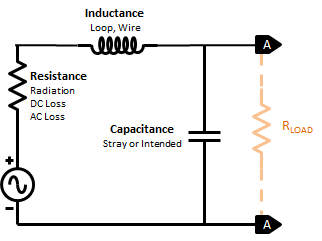Home » Radio » Shortwave Radio » Magnetic Loop Equivalent Circuit

Magnetic Loop Equivalent CircuitYour magnetic loop equivalent circuit is the engine under the hood for simulating the performance of an entire wideband active loop antenna. Here’s what it looks like.

Wouldn’t it be nice if you could just insert a chunk of aluminum into a an electronic design simulator and let it go to work? Life does not work that way. You need to describe your magnetic loop as some sort of electronic circuit that SPICE software will understand. This calls for a magnetic loop equivalent circuit, shown above.

An equivalent circuit is a behavioral model of loop hardware. It contains resistance, inductance and capacitance. To do simulation, you need to write the formulas that let the equivalent circuit mimic the actual circuit, which is a one meter diameter loop of half inch aluminum. Once you understand the basics, this is not all that hard to accomplish.

So, a SPICE model for a loop antenna needs an equivalent circuit that captures the resistance, inductance and capacitance of the mechanical structure.

To perform properly, the equivalent circuit must take into account three types of resistance. DC loss is the simplest, it’s just the resistance of the conductor. Both AC loss (skin effect) and radiation resistance are more complex, as they vary according to frequency. Inductance depends on the  parameters of the loop, in particular material, loop and conductor diameters, and number of turns. In addition, stay inductance arises from any length of wire, regardless of shape. Finally, any loop or conductor generates stray or distributed capacitance which is perhaps unintended but real. Intended capacitance may be added to resonate the loop purposely. Otherwise, the stray capacitance creates self-resonance.

When you connect the loop equivalent circuit to another circuit, such as a transmission line or amplifier, you place a load across the output (AA). A very small impedance load effectively shorts out the capacitance and enables wideband operation using loop current. Alternatively, a very high impedance load provides high output voltages at the resonant frequency, which is how tuned loops work.

Magnetic Loop Equivalent Circuit References

If you want to dig deeper, here are the key references that helped me understand this stuff. While I will keep my articles at the intermediate level, these references provide the heavy lifting.

This site uses Akismet to reduce spam. Learn how your comment data is processed.